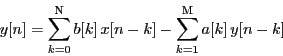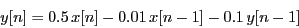# IIR Filters

Infinite Impulse Response filters are those filters present a non-zero infinite length response when excited with a very brief (ideally an infinite peak) input signal. A linear causal IIR filter can be described by the following difference equationThis operation describe a recursive system, i.e. a system that depends on current and past samples of the input x[n], but also on the output data stream y[n].

## Creating a IIR filter in the LTPDA

The LTPDA Toolbox allows the implementation of IIR filters by means of the miir class.

## Creating from a plist

The following example creates an order 1 highpass filter with high frequency gain 2. Filter is designed for 10 Hz sampled data and has a cut-off frequency of 0.2 Hz.

```
pl = plist('type', 'highpass', ...
'order', 1,         ...
'gain',  2.0,       ...
'fs',    10,        ...
'fc',    0.2);
f = miir(pl)
```

## Creating from a pzmodel

IIR filters can also be created from a pzmodel .

## Creating from a difference equation

Alternatively, the filter can be defined in terms of two vectors specifying the coefficients of the filter and the sampling frequency. The following example creates a IIR filter with sampling frequency 1 Hz and the following recursive equation:```
a = [0.5 -0.01];
b = [1 0.1];
fs = 1;
f = miir(a,b,fs)
```

Notice that the convetion used in this function is the one described in the Digital filters classification section

## Importing an existing model

The miir constructor also accepts as an input existing models in different formats:

• LISO files:

```      f = miir('foo_iir.fil')
```
• XML files:

```    f = miir('foo_iir.xml')
```
• MAT files:

```      f = miir('foo_iir.mat')
```
• From repository:

```      f = miir(plist('hostname', 'localhost', 'database', 'ltpda', 'ID', []))
```